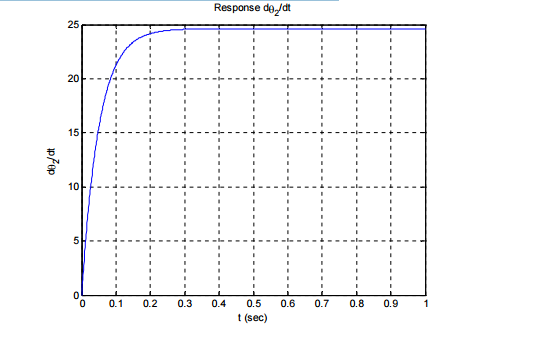# Back EMF in a Simple DC Motor

This is part of the HSC Physics syllabus under the topic Applications of the Motor Effect.

### HSC Physics Syllabus

• investigate the operation of a simple DC motor to analyse:

- the functions of its components

- production of a torque \tau = nIAB_(_|_) sin \theta

- effects of back emf (ACSPH108)

### What is Back EMF?

This video investigates the operation of a simple DC motor to analyse the effects of back emf.

### How Does a Simple DC Motor Produces Back EMF?

As the armature rotates within the external magnetic field, the amount of magnetic flux passing through the coil changes. This is because whilst the field strength is constant, the angle between the surface’s normal (area vector) and the flux lines changes. This causes the coil to experience an induced emf according to Faraday's Law.

Lenz's law states that the current associated with this induced emf will produce a magnetic field that opposes the external magnetic field. Thus, the induced emf’s direction is always opposite to the battery's supply voltage This is why this emf is called back emf.

As such, the net voltage of a DC motor can be described by:

$$V_{net} = V_{supply} - \varepsilon_{back}$$

As the coil's angular velocity increases, the rate of change in flux increases, which increases the back emf as \varepsilon = -N (∆\Phi)/(∆t). This means that the net voltage will decrease, causing the current to also decrease, according to Ohm's Law (V = IR). Since \tau = nIAB \sin \theta, a decreasing current (I) causes torque to decrease.

Eventually, the back emf will equal the supply voltage and the net voltage and current will equal 0. At this point, the coil has reached it's maximum rotational velocity since torque will be 0 N m. This is why back emf is said to limit the angular speed of a DC motor.

The graph below illustrates this phenomenon. The vertical axis is the angular velocity and the horizontal axis is time.Practice Question 1

(a) Describe the components that make up a DC motor (3 marks)

(b) Explain how the components you described in (a) achieve the function of a DC motor (2 marks)

Practice Question 2

A rectangular wire loop is connected to a DC power supply. Side X of the loop is placed next to a magnet. The loop is free to rotate about a pivot.

###When the power is switched on, a current of 20 A is supplied to the loop. To prevent rotation, a mass of 40 g can be attached to either side X or side Y of the loop.

(a) On which side of the loop should the mass be attached to prevent rotation? (1 mark)

(b) Calculate the torque provided by the 40 g mass. (2 marks)

(c) Calculate the magnetic field strength around side X. (3 marks)

Previous section: Parallel vs Radial Magnets

Next section: Operation of Simple DC and AC Generators